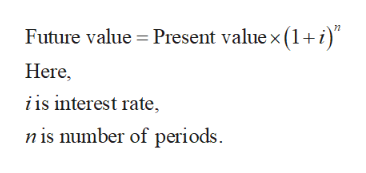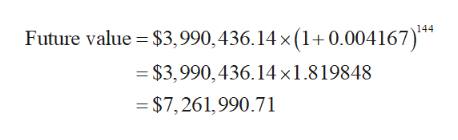# What would my total be earning 5% compounded monthly for 12 years after that (without any additional deposits) on \$3,990,436.14?

Question
6 views

What would my total be earning 5% compounded monthly for
12 years after that (without any additional deposits) on \$3,990,436.14?

check_circle

Step 1

Future value can be defined as the future worth of specified present amount at a future date at given interest rate.

It is given that,

Present value is \$3,990,436.14.

Interest rate is 5% compounded monthly. So, interest rate per month will be (0.05 ÷ 12) = 0.004167.

Number of years is 12. So, total number of months will be 12 × 12 =144.

Step 2

The formula to calculate future value is given below:help_outlineImage TranscriptioncloseFuture value Present value x (1+i Here, i is interest rate is number of periods fullscreen
Step 3

Substitute \$3,990,436.14 for present value, 0.004...help_outlineImage TranscriptioncloseFuture value \$3,990, 436.14 x (1+ 0.004167)* = \$3,990,436.14 x 1 .8 19848 = \$ 7, 261,990.71 fullscreen

### Want to see the full answer?

See Solution

#### Want to see this answer and more?

Solutions are written by subject experts who are available 24/7. Questions are typically answered within 1 hour.*

See Solution
*Response times may vary by subject and question.
Tagged in